# Does it matter what order the letters in an angle is written in? how does Prof. Loh's exterior angle strategy work?

• Module 2 Week 2 Day 6 Challenge Explanation Part 1

Does it matter what order an angle is written in? Like say angle ABC is 80 degrees. Would the number of degrees in the angle be different if it was BAC? What point is the vertex and how can you tell?

• Also at around timestamp 2:10 how does Prof. Loh know that in an isoceles triangle, if we know one angle's measure, we know the measures of all the other ones? How does that work?

• And one last question... At around timestamp 4:10, how does Prof. Loh's exterior angle strategy work?

• Hi TSS Graviser!

That's a rather deep question! First I'll clarify that the middle letter refers to the vertex of an angle, so it really does matter what order the letters are written, but not to the extent that it would seem, as in, it's not true that

$$\angle BAC \neq \angle ABC \neq \angle ACB \neq \angle BCA \neq \angle CBA \neq \angle CAB$$

(** Did you notice there were six ways to mix up these three letters? Remember that from Module 0?)

Some of these angles are the same (and to make it a little easier, please suppose we're talking about the interior angles of a triangle). For example,

\begin{aligned} \angle BAC &= \angle CAB \\ \angle CBA &= \angle ABC \\ \angle ACB &= \angle BCA \end{aligned}

What do the same angles have in common? They have the same middle letter!

So, there are three different letters that can be in the middle, and there are three different angles made using the letters $$A, B,$$ and $$C!$$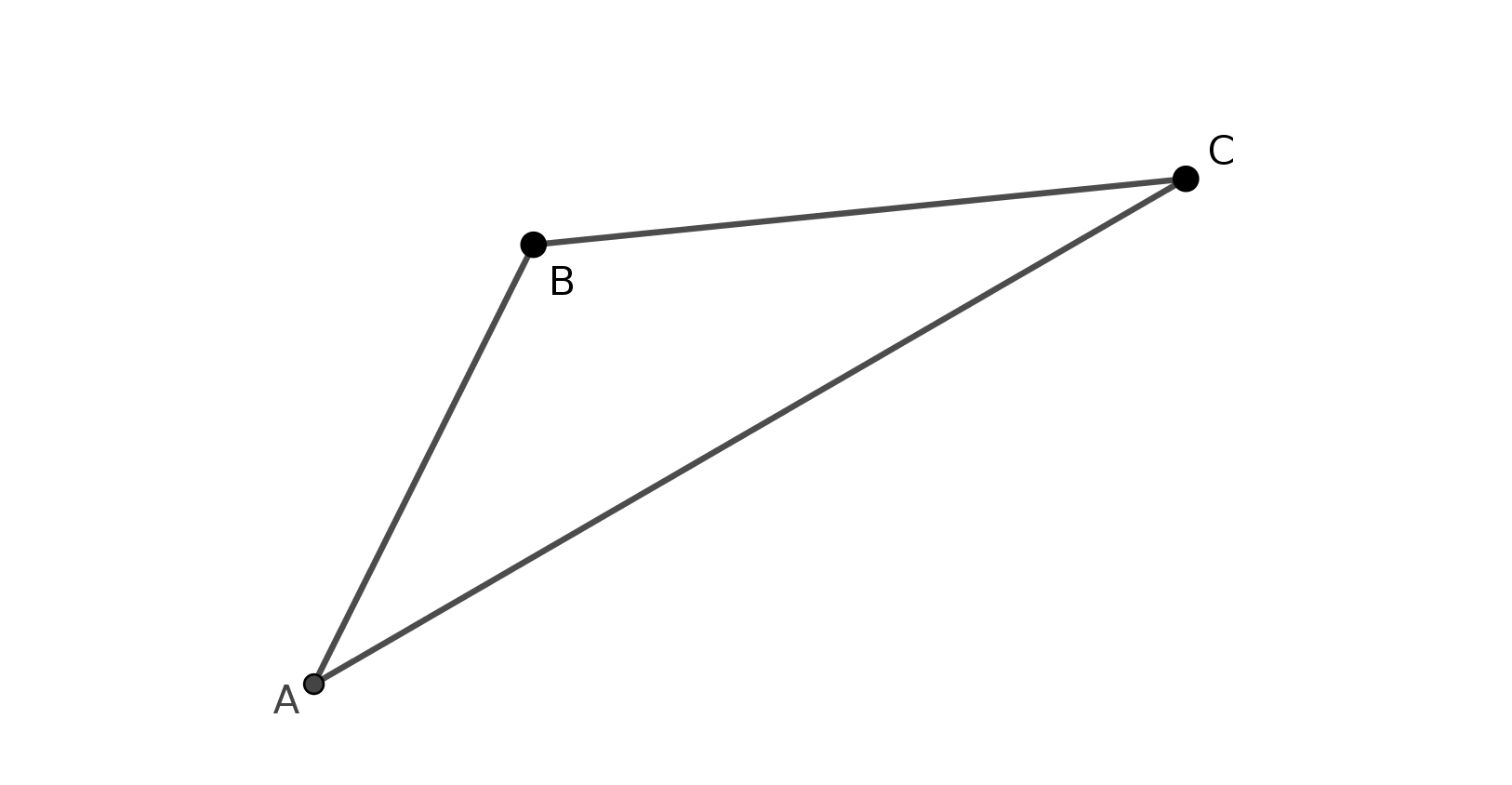But here's the deeper part: Even though we usually would write the angles in the way mentioned above, and refer to them like so naturally, there are actually six different angles. Why is that? Well, it's because for each angle, we can choose the "obtuse" ( > $$90^{\circ}$$ ) or "acute" (<$$90^{\circ}$$) angle.

For example, in this picture, both the purple and yellow angle are considered to be $$\angle CBA:$$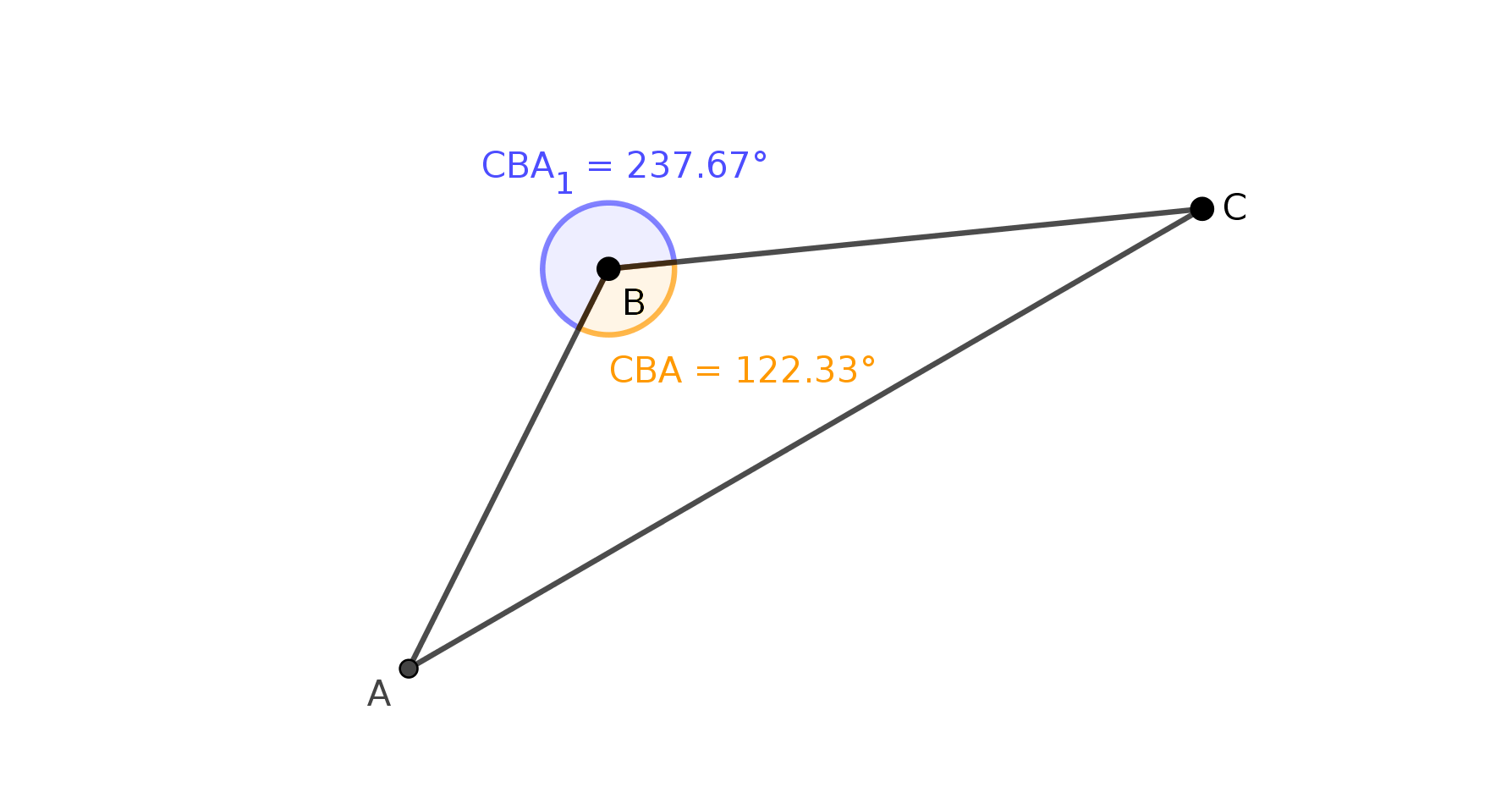And in this picture, both the purple and yellow angles are considered to be $$\angle BAC,$$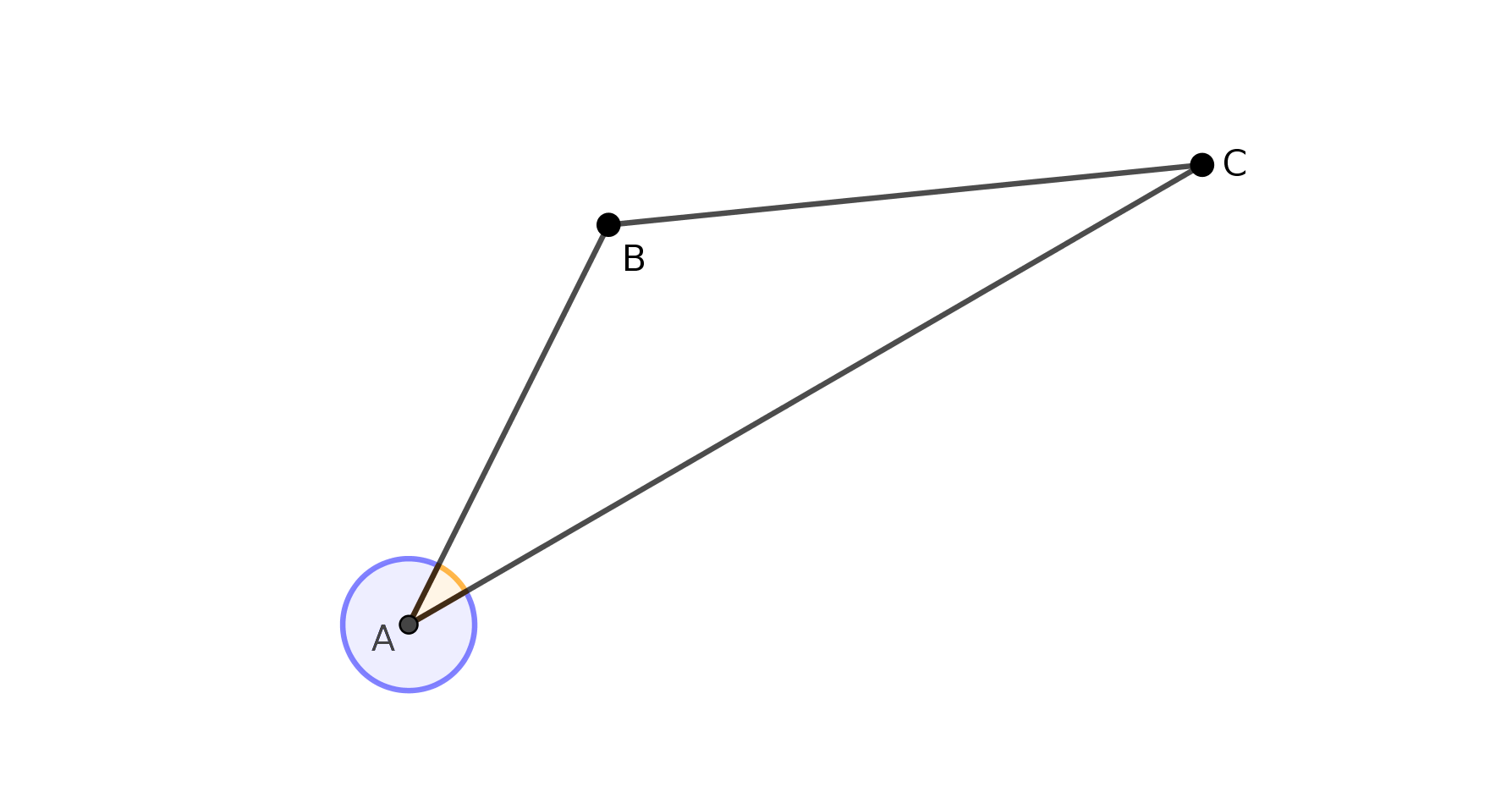And in this last picture, both the purple and yellow angles are considered to be $$\angle BCA:$$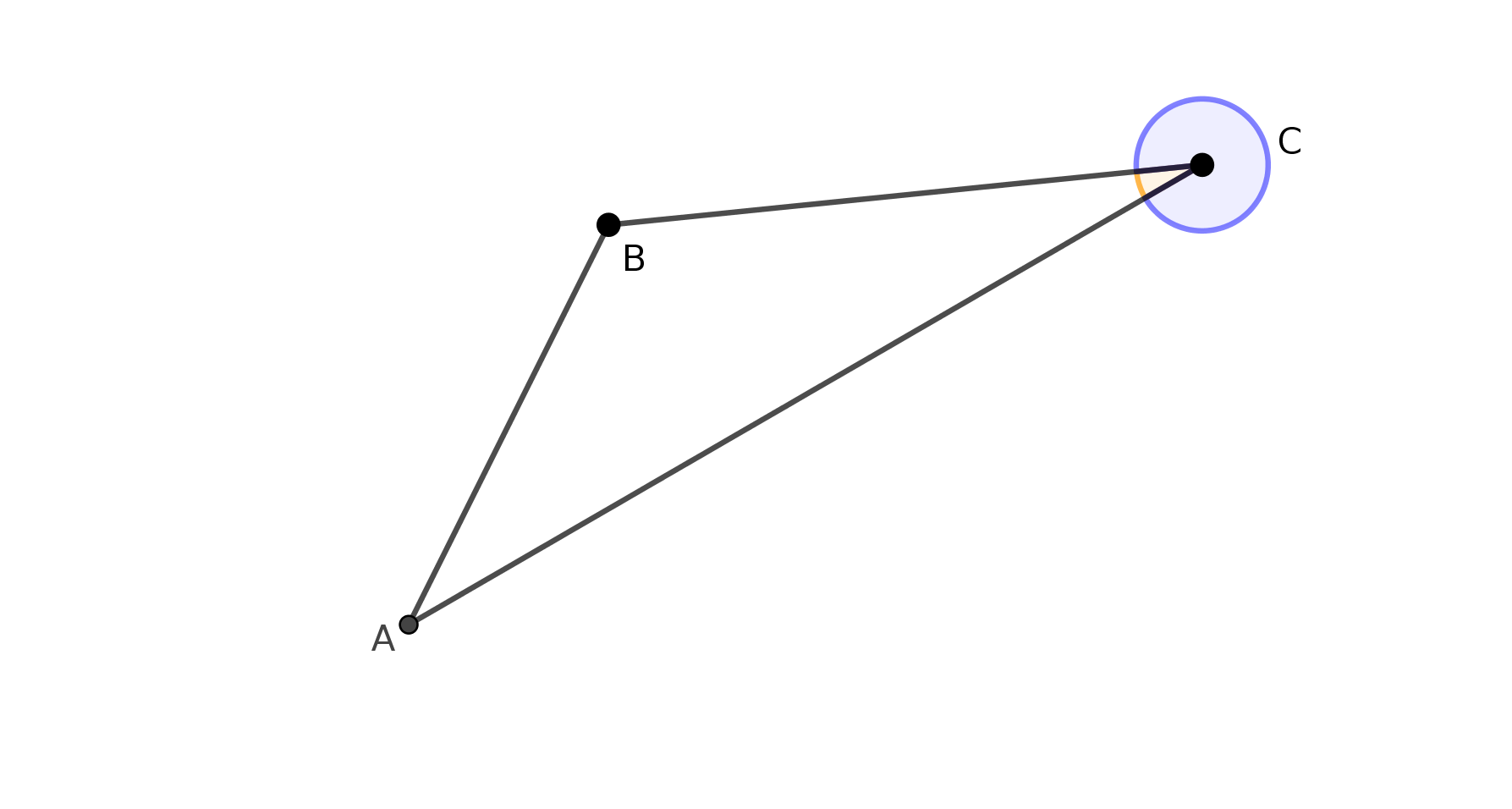I hope this makes it a little bit more clear! As long as you remember that the middle letter is the corner of the angle, then you'll be fine!

Happy Learning!

• @TSS-Graviser If we have an isosceles triangle like this, for example: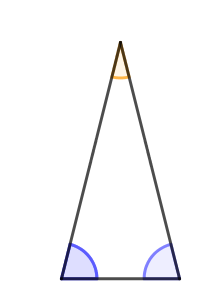we know that two of its angles are the same, because that's what makes an isosceles triangle an isosceles triangle.

Since the angles of a triangle add up to $$180^{\circ},$$ we know that

$$\angle \text{yellow} + \angle \text{purple} + \angle \text{purple} = 180^{\circ}$$

So if we knew the value of the angle $$\angle \text{yellow}$$ was equal to $$15^{\circ},$$ for example, we could write
$$15^{\circ} + \angle \text{purple} + \angle \text{purple} = 180^{\circ}$$

and we could solve for the value of the purple angle!

Conversely, if we knew that the purple angle was equal to $$80^{\circ},$$ we could write

$$\angle \text{yellow} + 80^{\circ} + 80^{\circ} = 180^{\circ}$$

and we could solve for the value of the yellow angle!

Happy Learning!

• @TSS-Graviser Let's look at the little triangle that Prof. Loh drew in the upper-right hand corner, and for the angle that he labeled with green marker, let's call that $$\angle \text{green}$$ for now. For the exterior angle, labeled with yellow marker, let's call that $$\angle \text{yellow}$$ for now.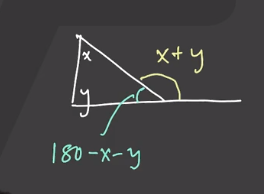Using the fact that the angles of a triangle add up to $$180^{\circ},$$ we can write

$$\angle x + \angle y + \angle \text{green} = 180^{\circ}$$

And, using the fact that $$\angle \text{green}$$ and $$\angle \text{yellow}$$ make up a line together,

$$\angle \text{green} + \angle \text{yellow} = 180^{\circ}$$

Or maybe I should write that with the angles in a different order:

$$\angle \text{yellow} + \angle \text{green} = 180^{\circ}$$

Do you know why I switched the order around? It's because now the first and third equations look very similar. They both have

$$\text{something} + \angle \text{green} = 180^{\circ}$$

So the two "somethings" are the same!

$$\angle x + \angle y = \angle \text{yellow}$$

That is the Exterior Angle Theorem, but the neat idea is that you don't have to memorize it, because you can instead think about exactly why it is true.

Happy Learning!

• But how do the isosceles triangles work the same way? They look very different from the right triangle above.

• @TSS-Graviser Yes, the isosceles triangle does look very different, but the neat thing about the underlying math here is that it doesn't matter what the triangles look like! This proof of the Exterior Angle Theorem just uses the fact that any triangle's angles add up to $$180^{\circ}.$$ Whether it is acute, obtuse, right, isosceles, equilateral... every triangle's angles add up to $$180^{\circ}.$$

The angles that make up a line add up to $$180^{\circ}$$ as well, and this is the reason behind the Exterior Angle Theorem. The sum of the interior angles of the triangle equals the sum of the angles on the line drawn by extending one of the triangle's sides.